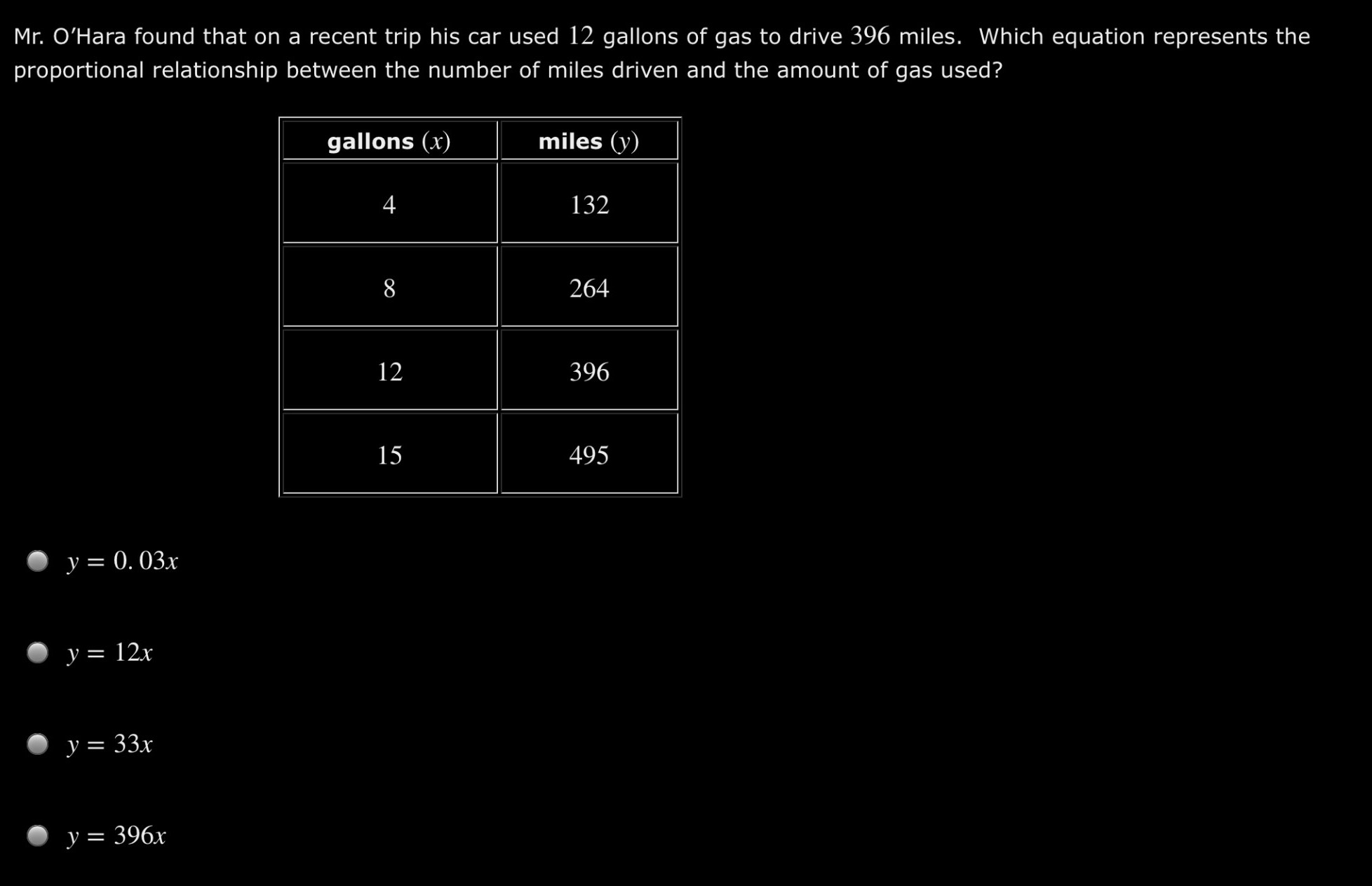### Still have math questions?

Algebra
QuestionMr. O'Hara found that on a recent trip his car used $$12$$ gallons of gas to drive $$396$$ miles. Which equation represents the proportional relationship between the number of miles driven and the amount of gas used?

$$y = 0.03 x$$

$$y = 12 x$$

$$y = 33 x$$

$$y = 396 x$$